### Home > PC > Chapter 1 > Lesson 1.1.2 > Problem1-24

1-24.
1. Suppose f(x) = 2x2 − 3, find: Homework Help ✎

1. f(4)

2. f(−5)

3. f(3b)

4. f(a + 1)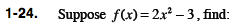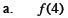Substitute 4 for every x in the function.

f(4) = 2(4)2 − 3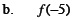Use ( ) when substituting to avoid errors.

f(−5) = 2(−5)2 − 3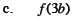Follow the same steps for (b).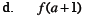Do not forget the middle term when expanding (a + 1)2.

2a2 + 4a − 1